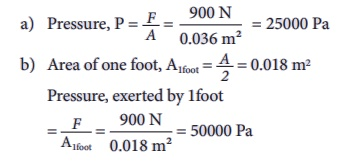Home | | Science 9th std | Thrust and Pressure

# Thrust and Pressure

The net force in a particular direction is called thrust. The force per unit area acting on an object concerned is called pressure. Thus, we can say thrust on an unit area is pressure.

Thrust and Pressure

Try to fix a paper with the help of a drawing pin. Push the pin into the board by its head. Did you succeed? Try now to push the pin by the pointed end. Could you do it this time? Have you ever wondered why a camel can run in a desert easily? Why a truck or a motorbus has wider tyre? Why cutting tools have sharp edges? In order to answer these questions and understand the phenomena involved, we need to learn about two interrelated physical concepts called thrust and pressure.

In both the cases of the above activity, the force exerted on the sand is the weight of your body which is the same. This force acting perpendicular to the surface is called thrust. When you stand on loose sand, the force is acting on an area equal to the area of your feet. When you lie down, the same force acts on an area of your whole body, which is larger than the area of your feet. Therefore the effect of thrust, that is, pressure depends on the area on which it acts. The effect of thrust on sand is larger while standing than lying.

The net force in a particular direction is called thrust. The force per unit area acting on an object concerned is called pressure. Thus, we can say thrust on an unit area is pressure.

Pressure = Thrust/Area of contactFor the same given force, if the area is large pressure is low and vice versa. This is shown in Figure 1.1.In SI units, the unit of thrust is newton denoted as N. The unit of pressure is newton per square metre or newton metre–2 denoted as Nm–2. In the honour of the great French scientist, Blaise Pascal, 1 newton per square metre is called as 1 pascal denoted as Pa. 1 Pa = 1 N m–2

In CGS system force is measured in dyne and area in square cm. Thus the unit of pressure in CGS system is dyne per square cm (dyne cm-2). The relation between the two units is,

1 N m–2 = 10 dyne cm–2.

### Example 1.1

A man whose mass is 90 kg stands on his  feet on a floor. The total area of contact  of his two feet with the floor is 0.036 m2.  (Take, g = 10 ms–2)

a. How much is the pressure exerted by him on the floor?

b. What pressure will he exert on the floor if he stands on one foot?

### Solution

The weight of the man (thrust),

F = mg = 90 kg × 10 ms–2 = 900 NStudy Material, Lecturing Notes, Assignment, Reference, Wiki description explanation, brief detail
9th Science : Fluids : Thrust and Pressure |